# The proof - math problems

The proof is a convincing demonstration in mathematics that a statement is true under certain conditions.

#### Number of problems found: 15

• WorkersThree factory operators produced 480 units in 50 minutes. How many hours worked? I'm trying to prove or disapprove the idea that the company tell me that it's 2.5 hours. So what is right? Thank you, Petra
• Odd/even numberPick any number. If that number is even, divide it by 2. If it's odd, multiply it by 3 and add 1. Now repeat the process with your new number. If you keep going, you'll eventually end up at 1. Every time. Prove. ..
• ProveProve that k1 and k2 are the equations of two circles. Find the equation of the line that passes through the centers of these circles. k1: x2+y2+2x+4y+1=0 k2: x2+y2-8x+6y+9=0
• Sum of inner angles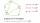Prove that the sum of all inner angles of any convex n-angle equals (n-2) . 180 degrees.
• Equilateral cylinder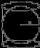A sphere is inserted into the rotating equilateral cylinder (touching the bases and the shell). Prove that the cylinder has both a volume and a surface half larger than an inscribed sphere.
• Three numbers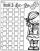How much we increases the sum of three numbers when the first enlarge by 14, second by 15 and third by 16? Choose any three two-digit numbers and prove results.
• Diagonal in rectangle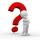In that rectangle ABCD is the center of BC point E and point F is center of CD. Prove that the lines AE and AF divide diagonal BD into three equal parts.
• Theorem proveWe want to prove the sentence: If the natural number n is divisible by six, then n is divisible by three. From what assumption we started?
• Truncated coneCalculate the height of the rotating truncated cone with volume V = 794 cm3 and a base radii r1 = 9.9 cm and r2 = 9.8 cm.
• See harmonics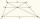It is true that the size of the central segment of any trapezoid is the harmonic mean size of its bases? Prove it. Central segment crosses the intersection of the diagonals and is parallel to the bases.
• Proof PTCan you easily prove Pythagoras theorem using Euclidean theorems? If so, do it.
• TriangleProve whether you can construct a triangle ABC, if a=9 cm, b=6 cm, c=10 cm.
• Reciprocal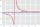It is true (prove it) that if a> b> 0: ?
• Proof I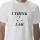When added to the product of two consecutive integers larger one, we get square larger one. Is this true or not?
• Engineer KažimírThe difference between politicians-demagogues and reasonable person with at least primary education beautifully illustrated by the TV show example. "Engineer" Kažimír says that during their tenure there was a large decline in the price of natural gas, pri

Do you have an exciting math question or word problem that you can't solve? Ask a question or post a math problem, and we can try to solve it.

We will send a solution to your e-mail address. Solved examples are also published here. Please enter the e-mail correctly and check whether you don't have a full mailbox.

Please do not submit problems from current active competitions such as Mathematical Olympiad, correspondence seminars etc...